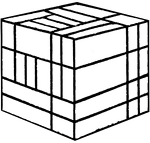### Froebel's Divided Cube (Complex)

This image shows one of Friedrich Froebel's divided cube (this one divided into many smaller cubes and…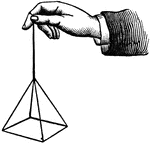### Hand and pyramid

A hand holding a square pyramid, suspended by a string.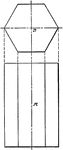### Projection of Hexagonal Bar

Projection of a hexagonal bar.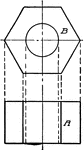### Projection of Hexagonal Nut

Projection of a hexagonal nut.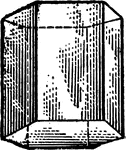### Hexagonal Prism

"Science has succeeded in classifying the thousands of known crystals in six systems, to each of which…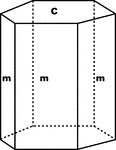### Hexagonal Prism and Basal Pinacoid

"...shows a combination of a hexagonal prism (m) with the basal pinacoid (c)." -The Encyclopedia Britannica…### Hexagonal Prism of the First Order

"Hexagonal prism of the first order, consisting of six faces also parallel to the hexad axis, but perpendicular…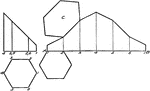### Development of Hexagonal Prism

An illustration of a hexagonal prism rolled out, or "stretched out" in the straight line AB.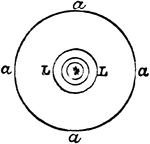"Holophotal Catadioptric Apparatus Revolving round a Central Flame." —The Encyclopedia Britannica,…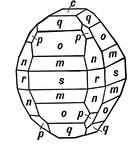### Hydrous Nickel Sulphate

This form shows the planes given on a crystal of hydrous nickel sulphate: the basal pinacoid, (c); three…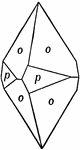Also known as Stolzite, this crystal is bounded by the unit pyramid of the first order, P, {111} (o),…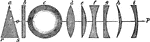### Lenses of Various Forms

"a, prism; b, plane glass; c, spherical lens; d, double-convex; e, plano-convex, f, double-concave;…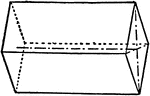### Macro-prism and Brachy-pinacoid

"This consists of four faces parallel to the brachy axis." -The Encyclopedia Britannica 1910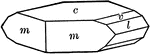### Marcasite

"Orthorhombic. Crystals commonly tabular parallel to basal plane, showing also short prisms and low…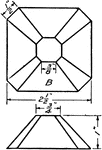### Development Exercise of Octagonal Light Shade

A problem exercise creating a stretched out or developed image of the octagonal light shade by using…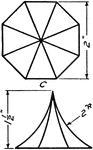### Development Exercise of Octagonal Roof and True Shape of Rafter

Problem exercise in drawing a development or a stretchout image of the octagonal roof and finding the…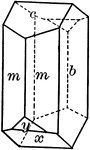### Orthoclase

"Monoclinic. Crystals are usually prismatic in habit and have as prominent forms, clinopinacoid, base,…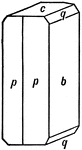### Orthorhombic Crystal of Olivine

This is an orthorhombic crystal of olivine. It is formed by the orthorhombic prism in combination with…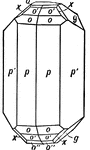### Orthorhombic Crystal of Topaz

This form shows the fundamental prism (p) on a crystal of topaz, in combination with another prism,…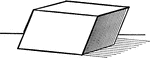### Parallelopiped

Illustration of a parallelopiped - a prism with a parallelogram as its base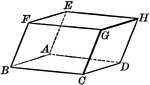### Parallelopiped

Illustration of a parallelopiped - a prism with a parallelogram as its base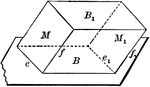### Equal and Parallel Opposite Faces of a Parallelopiped

Diagram used to prove the theorem: "The opposite faces of a parallelopiped are equal and parallel."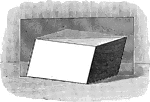### Parallelopiped with Plane Passing Through

Illustration of a parallelopiped with a plane passing through two diagonally opposite edges. The plane…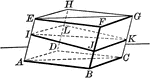### Parallelopiped with Plane Passing Through

Illustration of a parallelopiped with a plane passing through two diagonally opposite edges. The plane…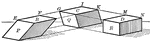### Parallelopiped Showing Volume

Illustration of a parallelopiped (a prism with a parallelogram as its base) used to demonstrate that…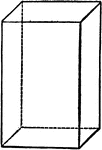### Parallelopipedon

"A parallelopipedon is a prism whose bases (ends) are parallelograms." —Hallock 1905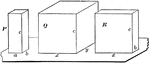### Relationship Between 2 Parallelopipeds With Equal Altitudes

Diagram used to prove the theorem: "The rectangular parallelopipeds which have two dimensions in common…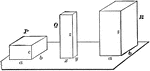### Relationship Between Dimensions of Parallelopipeds

Diagram used to prove the theorem: "The rectangular parallelopipeds are to each other as the product…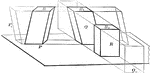### Volume of Parallelopiped

Diagram used to prove the theorem: "The volume of a any parallelopiped is equal to the product of its…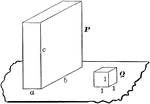### Volume of Rectangular Parallelopiped

Diagram used to prove the theorem: "The volume of a rectangular parallelopiped is equal to the product…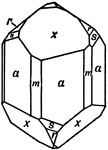### Phenacite

This crystal is mainly terminated by a rhombohedron of the third order, (x). With this form are associated…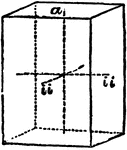### Primitive Crystal

"If the base is a square and the prism stands erect—that is, if its sides or lateral planes, as…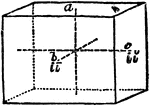### Primitive Crystal

"When the base is a rectangle instead of a square, the form is a right rectangular prism." —The…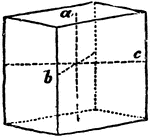### Primitive Crystal

"When the base is a rhombus, and the prism stands erect, the form is a right rhombic prism." —The…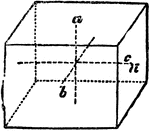### Primitive Crystal

"When the base is a rhomboid, and the prism stands erect, it is only the opposite laeral faces that…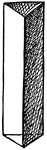### Prism

A transparent body, with usually three sides and two equal and parallel triangular ends or bases.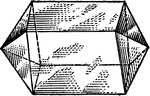### Prism

"Right rhombic prism." — Hallock, 1905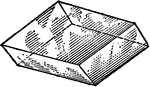### Prism

"Doubly oblique prism." — Hallock, 1905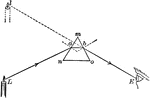### Prism

"A prism is a transparent body with two refraction surfaces that lie in intersecting planes. The angle…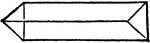### Prism

"A prism is a piece of glass, having for its sides three plane surfaces and for its ends two equal and…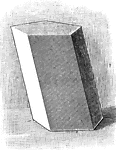### Prism

Illustration of a prism - a polyhedron of which two faces are equal polygons in parallel planes, and…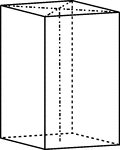### Prism and Basal Pinacoid

Holohedral orthorhombic combination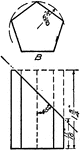### Pentagon Development Prism Exercise

An exercise developed to draw a rolled out or development of the pentagon prism in a 4" by 5" surface.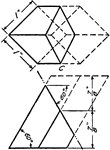### Pentagonal Prism Development Exercise

An exercise in drawing a pentagonal prism development or rolled out image in a 4" by 5" area.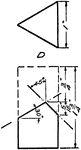### Triangular Prism Development Exercise

An illustration to exercise a stretched out, or development, image of the triangular prism using 4"…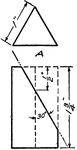### Triangle Prism Exercise

An illustration to draw triangle prism's development, or stretched out surfaces, in a 4" by 5" surface.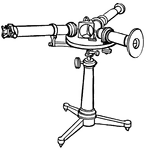### Prism Spectroscope from the Late 19th Century

"...a tube with a slit at the further end through which the light enters, and at the other end a collimating…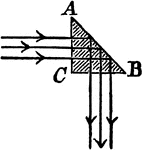### Cathetal Prism

"Cathetal prisms readily yield the phenomena of total reflection as shown, and are often used when light…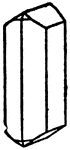### Combination of Prisms

An illustration of a combination formed by different prisms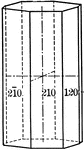### Ditetragonal prism

"The dietetragonal prism is a form consisting of eight rectangular vertical faces, each of which intersects…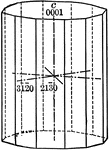### Dihexagonal prism

"The dihexagonal prism has twelve rectangular vertical faces, each of which intersects all three of…### First order prism

"The prism of the first order consists of four rectangular vertical faces, each of which intersects…### Hexagonal Prism

Prism with hexagonal bases and dimensions 8 and 12 given.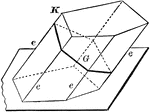### Lateral Area of A Prism

Diagram used to prove the theorem: "The lateral area of a prism is equal to the product of a lateral…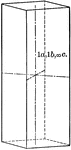### Orthohombie prism

"Crystal faces are described according to their relations to the crystallographic axes. A series of…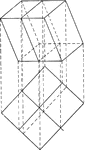### Intersection of a Prism and a Plane

Illustration of the intersection of a prism and a plane.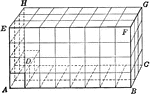### Rectangular Prism

Prism with rectangular bases and cubes drawn in.### Right Triangular Prism

Prism with triangular bases.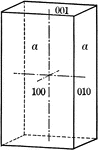### Second order prism

"The prism of the second order consists of four rectangular vertical faces, each of which intersects…